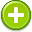#equivalent 中文解釋 wordnet sense Collocation Usage
/iˈkwivələnt/,Font size:Equal in value, amount, function, meaning, etc,
1. Equal in value, amount, function, meaning, etc
• - one unit is equivalent to one glass of wine
2. Having the same or a similar effect as
• - some regulations are equivalent to censorship
3. Belonging to the same equivalence class

Noun
1. A person or thing that is equal to or corresponds with another in value, amount, function, meaning, etc
• - the French equivalent of the FBI
2. The mass of a particular substance that can combine with or displace one gram of hydrogen or eight grams of oxygen, used in expressing combining powers, esp. of elements

1. a person or thing equal to another in value or measure or force or effect or significance etc; "send two dollars or the equivalent in stamps"
2. being essentially equal to something; "it was as good as gold"; "a wish that was equivalent to a command"; "his statement was tantamount to an admission of guilt"
3. the atomic weight of an element that has the same combining capacity as a given weight of another element; the standard is 8 for oxygen
4. (equivalence) equality: a state of being essentially equal or equivalent; equally balanced; "on a par with the best"
5. (equivalence) essential equality and interchangeability
6. (equivalence) comparison: qualities that are comparable; "no comparison between the two books"; "beyond compare"
7. The equivalent (symbol: eq or Eq), sometimes termed the molar equivalent, is a unit of amount of substance used in chemistry and the biological sciences.
8. In computability theory a numbering is the assignment of natural numbers to a set of objects like rational numbers, graphs or words in some language. ...
9. The Equivalent was a sum negotiated at £398,000 paid to Scotland by the English Government under the terms of the Acts of Union 1707. Proposals for it first emerged in the course of abortive Union negotiations in 1702/03.
10. Equivalents is a series of photographs of clouds taken by Alfred Stieglitz from 1925 to 1934. They are generally recognized as the first photographs intended to free the subject matter from literal interpretation, and, as such, are some of the first completely abstract photographic works of art.
11. (Equivalence (category theory)) In category theory, an abstract branch of mathematics, an equivalence of categories is a relation between two categories that establishes that these categories are "essentially the same". ...
12. (Equivalence (measure theory)) In mathematics, and specifically in measure theory, equivalence is a notion of two measures being "the same".
13. anything that is virtually equal to another; an equivalent weight; similar or identical in value, meaning or effect; virtually equal; of two sets, having a one-to-one relationship; relating to the corresponding elements of an equivalence relation; having the equal ability to combine; of a ...
14. (Equivalently) the scale-space family can be defined as the solution of the diffusion equation (for example in terms of the heat equation),
15. (equivalence) In the context of text processing, the process or result of establishing whether two text elements are identical in some respect.
16. (Equivalence) A truth function that returns truth when its two arguments have the same truth-value, and false otherwise. Also the connective denoting this function; also the compound proposition built from this connective. Syntactically: the two propositions imply one another. ...
17. (Equivalence) The number of protons donated in an acid-base reaction or the total change in valence in an oxidation-reduction reaction.
18. (Equivalence) The property of a map projection to represent all areas in true proportion to one another. See Chapter 6.
19. (Equivalence) The relationship between preferred and non-preferred terms where two or more terms are regarded, for indexing purposes, as referring the same concept. Relationship linking non-preferred terms to preferred terms.
20. (Equivalence) the same intension (empirical case) or the same object (mathematical case)
21. (equivalence) A way of saying that two things are treated the same according to a definition of equality. In sensemaking, notions of equivalence are important for governing retrieval based on assumed similarity of meaning. ...
22. (equivalence) The ratio of antibody to antigen at which immunoprecipitation of the reactants is virtually complete.
23. (equivalence) Two lots of product are equivalent if, within experimental error, they are essentially equal in purity/impurity, potency, identity, and safety. A more stringent requirement than comparability. (See comparable)
24. (equivalence) in logic, a relationship between two statements such that either both are true or neither is.
25. (equivalence) the recognizable identity of the reality experienced and symbolized on various levels of differentiation